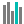#BINOM.DIST.RANGE (PQL - xl)

This function uses binomial distribution to calculate the probability of a trial result

• Library: PQL \ Spreadsheet \ Statistical
• Compatibility: Any content (regardless of data source) in the Tabulate spreadsheet module

#### Syntax

BINOM.DIST.RANGE(trials, probability s, number s, number s2)

##### Function Arguments
 Name Description Type Optional trials Refers to the number representing the number of independent trials. If this value is not an integer, the number is truncated Number probability s Refers to the probability of success on each trial. The value of this number must lie between 0 and 1 Number number s Refers to the number representing the number of successes in trials. If this value is not an integer, the number is truncated Number number s2 Refers to the probability that number of successful trials will fall between s and s2. This value must be greater than or equal to s and less than or equal to N Number